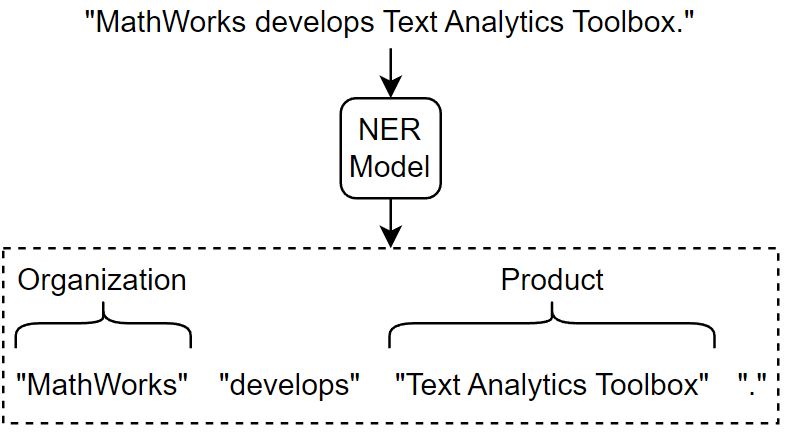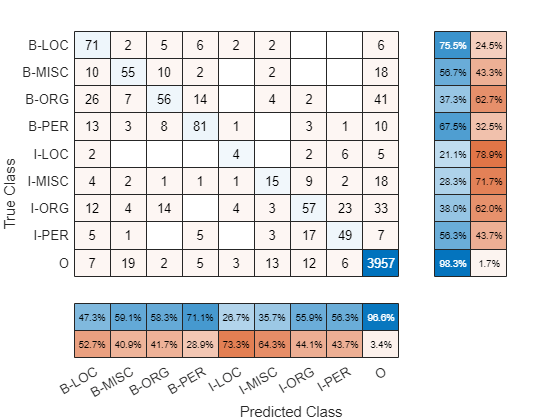# Train Custom Named Entity Recognition Model

This example shows how to train a custom named entity recognition (NER) model.

Named entity recognition (NER)  is the process of detecting named entities in text such as "person" or "organization". This diagram shows text flowing through a NER model.The `addEntityDetails` function automatically detects person names, locations, organizations, and other named entities in text. If you want to train a custom model that predicts different tags, or train a model using your own data, then you can use the `trainHMMEntityModel` function.

```dataFolder = fullfile(tempdir,"wikigold"); if ~datasetExists(dataFolder) zipFile = matlab.internal.examples.downloadSupportFile("textanalytics","data/wikigoldData.zip"); unzip(zipFile,dataFolder); end```

`filenameTrain = fullfile(dataFolder,"wikigold.conll.txt");`

The training data contains approximately 39,000 tokens, each tagged with one of these entities:

• `"LOC"` — Location

• `"MISC"` — Miscellaneous

• `"ORG"` — Organization

• `"PER"` — Person

• `"O"` — Outside (non-entity)

The entity tags use the "inside, outside" (IO) labeling scheme. The tag `"O"` (outside) denotes non-entities. For each token in an entity, the tag is prefixed with `"I-"` (inside), which denotes that the token is part of an entity.

A limitation of the IO labeling scheme is that it does not specify entity boundaries between adjacent entities of the same type. The "inside, outside, beginning" (IOB) labeling scheme, also known as the "beginning, inside, outside" (BIO) labeling scheme, addresses this limitation by introducing a "beginning" prefix.

There are two variants of the IOB labeling scheme: IOB1 and IOB2.

#### IOB2 Labeling Scheme

For each token in an entity, the tag is prefixed with one of these values:

• `"B-"` (beginning) — The token is a single token entity or the first token of a multi-token entity.

• `"I-"` (inside) — The token is a subsequent token of a multi-token entity.

For a list of entity tags `Entity`, the IOB2 labeling scheme helps identify boundaries between adjacent entities of the same type by using this logic:

• If `Entity(i)` has prefix `"B-"` and `Entity(i+1)` is `"O"` or has prefix `"B-"`, then `Token(i)` is a single entity.

• If `Entity(i)` has prefix `"B-"`, `Entity(i+1)`, ..., `Entity(N)` has prefix `"I-"`, and `Entity(N+1)` is `"O"` or has prefix `"B-"`, then the phrase `Token(i:N)` is a multi-token entity.

#### IOB1 Labeling Scheme

The IOB1 labeling scheme does not use the prefix `"B-"` when an entity token follows an `"O-"` prefix. In this case, an entity token that is the first token in a list or follows a non-entity token implies that the entity token is the first token of an entity. That is, if `Entity(i)` has prefix `"I-"` and `i` is equal to 1 or `Entity(i-1)` has prefix `"O-"`, then `Token(i)` is a single token entity or the first token of a multi-token entity.

Read the data from the training data using the `readWikigoldData` function, attached to this example as a supporting file. To access this function, open the example as a live script. The `trainHMMEntityModel` function requires data in the IOB2 labeling scheme. The function converts the tags from IO labeling scheme to the IOB2 labeling scheme.

`[data,numDocuments] = readWikigoldData(filenameTrain);`

View the number of documents.

`numDocuments`
```numDocuments = 145 ```

Partition the data into training and test sets. Set aside 10% of the documents for testing.

`cvp = cvpartition(numDocuments,HoldOut=0.1);`

Find the indices of the training and test documents.

```idxDocumentsTrain = find(training(cvp)); idxDocumentsTest = find(test(cvp));```

Find the tokens in the training data corresponding to the training and test documents.

```idxTokensTrain = ismember(data.DocumentNumber,idxDocumentsTrain); idxTokensTest = ismember(data.DocumentNumber,idxDocumentsTest);```

Partition the data using the token indices.

```dataTrain = data(idxTokensTrain,:); dataTest = data(idxTokensTest,:);```

View the first few rows of the training data. The table contains three columns that represent token indices, tokens, and the entities.

`head(dataTrain)`
``` Token Entity DocumentNumber __________ ________ ______________ "010" "B-MISC" 1 "is" "O" 1 "the" "O" 1 "tenth" "O" 1 "album" "O" 1 "from" "O" 1 "Japanese" "B-MISC" 1 "Punk" "O" 1 ```

View the entity tags.

`unique(dataTrain.Entity)`
```ans = 9×1 string "B-LOC" "B-MISC" "B-ORG" "B-PER" "I-LOC" "I-MISC" "I-ORG" "I-PER" "O" ```

### Train NER Model

Train the NER model and indicate that the tag `"O"` denotes non-entities. Depending on the size of the training data, this step can take a long time to run.

`mdl = trainHMMEntityModel(dataTrain,NonEntity="O")`
```mdl = hmmEntityModel with properties: Entities: [5×1 categorical] ```

### Test NER Model

View the first few rows of the validation data.

`head(dataTest)`
``` Token Entity DocumentNumber ____________ _______ ______________ "Albert" "B-PER" 18 "Wren" "I-PER" 18 "was" "O" 18 "an" "O" 18 "Ontario" "B-LOC" 18 "politician" "O" 18 "." "O" 18 "He" "O" 18 ```

Make predictions on the validation data using the trained model.

```documentsTest = tokenizedDocument(dataTest.Token',TokenizeMethod="none"); tbl = predict(mdl,documentsTest);```

View the first few predictions.

`head(tbl)`
``` Token Entity ____________ ______ "Albert" B-PER "Wren" I-PER "was" O "an" O "Ontario" B-LOC "politician" O "." O "He" O ```

Visualize the results in a confusion chart.

```YTest = string(tbl.Entity); TTest = dataTest.Entity; figure confusionchart(TTest,YTest,RowSummary="row-normalized",ColumnSummary="column-normalized")```Calculate the average F1-score using the `f1Score` function, listed in the F1-Score Function section of the example.

`score = f1Score(TTest,YTest)`
```score = 0.5634 ```

### Make Predictions with New Data

Create a tokenized document.

```str = [ "William Shakespeare was born in Stratford-upon-Avon, England." "He wrote plays such as Julius Caesar and King Lear."]; documentsNew = tokenizedDocument(str)```
```documentsNew = 2×1 tokenizedDocument: 9 tokens: William Shakespeare was born in Stratford-upon-Avon , England . 11 tokens: He wrote plays such as Julius Caesar and King Lear . ```

Add entity details to the documents using the `addEntityDetails` function and specify the trained model.

`documentsNew = addEntityDetails(documentsNew,Model=mdl);`

View the token details of the first few tokens. The `addEntityDetails` function automatically merges multi-token entities into a single token and removes the `"B-"` and `"I-"` prefixes from the tags.

`details = tokenDetails(documentsNew)`
```details=17×8 table Token DocumentNumber SentenceNumber LineNumber Type Language PartOfSpeech Entity _____________________ ______________ ______________ __________ ___________ ________ _________________ ______ "William Shakespeare" 1 1 1 other en proper-noun PER "was" 1 1 1 letters en auxiliary-verb O "born" 1 1 1 letters en verb O "in" 1 1 1 letters en adposition O "Stratford-upon-Avon" 1 1 1 other en proper-noun LOC "," 1 1 1 punctuation en punctuation O "England" 1 1 1 letters en proper-noun LOC "." 1 1 1 punctuation en punctuation O "He" 2 1 1 letters en pronoun O "wrote" 2 1 1 letters en verb O "plays" 2 1 1 letters en noun O "such" 2 1 1 letters en adjective O "as" 2 1 1 letters en adposition O "Julius Caesar" 2 1 1 other en proper-noun PER "and" 2 1 1 letters en coord-conjunction O "King Lear" 2 1 1 other en proper-noun PER ⋮ ```

Extract the `Token` and `Entity` variables of the table.

`details(:,["Token" "Entity"])`
```ans=17×2 table Token Entity _____________________ ______ "William Shakespeare" PER "was" O "born" O "in" O "Stratford-upon-Avon" LOC "," O "England" LOC "." O "He" O "wrote" O "plays" O "such" O "as" O "Julius Caesar" PER "and" O "King Lear" PER ⋮ ```

### F1-Score Function

The `f1Score` function takes as input a data set of targets and predictions and returns the F1-score averaged over the classes. This metric is suited for data sets with imbalanced classes. The metric is given by

`$\text{score}=\frac{2×\text{precision}×\text{recall}}{\text{precision}+\text{recall}},$`

where $\text{precision}$ and $\text{recall}$ denote the precision and recall averaged over the classes given by:

`$\begin{array}{l}\text{precision}=\frac{1}{K}\sum _{i=1}^{K}\frac{{\text{TP}}_{i}}{{\text{TP}}_{i}+{\text{FP}}_{i}},\\ \text{recall}=\frac{1}{K}\sum _{i=1}^{K}\frac{{\text{TP}}_{i}}{{\text{TP}}_{i}+{\text{FN}}_{i}},\end{array}$`

where $K$ is the number of classes, and ${\text{TP}}_{i}$, ${\text{FP}}_{i}$, and ${\text{FN}}_{i}$, denote the numbers of true positives, false positives, and false negatives for class $i$, respectively.

```function score = f1Score(targets,predictions) classNames = unique(targets); numClasses = numel(classNames); TP = zeros(1,numClasses); FP = zeros(1,numClasses); FN = zeros(1,numClasses); for i = 1:numel(classNames) name = classNames(i); TP(i) = sum(targets == name & predictions == name); FP(i) = sum(targets ~= name & predictions == name); FN(i) = sum(targets == name & predictions ~= name); end precision = mean(TP ./ (TP + FP)); recall = mean(TP ./ (TP + FP)); score = 2*precision*recall/(precision + recall); end```

### Bibliography

1. Krishnan, Vijay, and Vignesh Ganapathy. "Named entity recognition." Stanford Lecture CS229 (2005).

2. Dominic Balasuriya, Nicky Ringland, Joel Nothman, Tara Murphy, and James R. Curran. "Named entity recognition in wikipedia." In Proceedings of the 2009 workshop on the people’s web meets NLP: Collaboratively constructed semantic resources (People’s Web), pp. 10-18. 2009.